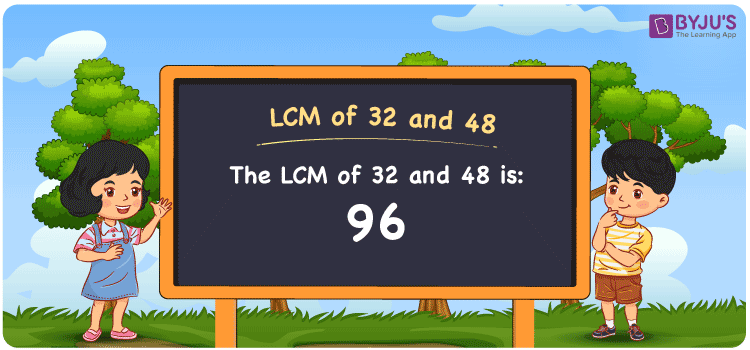Checkout JEE MAINS 2022 Question Paper Analysis : Checkout JEE MAINS 2022 Question Paper Analysis :

# LCM of 32 and 48

LCM of 32 and 48 is 96. The lowest common multiple of any two or more natural numbers is the number that is the lowest of their common multiples. Expert faculty designed the article HCF and LCM in simple language to help students solve the problems based on HCF and LCM effortlessly. This also helps students to improve problem-solving and time management skills, which are crucial from an exam point of view. Let us learn how to find the least common multiple of 32 and 48 with simple steps in this article.

## What is LCM of 32 and 48?

The Least Common Multiple of 32 and 48 is 96.## How to Find LCM of 32 and 48?

The LCM of 32 and 48 can be determined using the following methods:

• Prime Factorisation
• Division method
• Listing the multiples

### LCM of 32 and 48 Using Prime Factorisation Method

By prime factorisation method, we can write 32 and 48 as the product of prime numbers, such that;

32 = 2 × 2 × 2 × 2 × 2

48 = 2 × 2 × 2 × 2 × 3

LCM (32, 48) = 2 × 2 × 2 × 2 × 2 × 3 = 96

### LCM of 32 and 48 Using Division Method

In this method, we calculate the LCM by dividing the numbers 32 and 48 by their prime factors until we get the result as one in the complete row. The product of these divisors denotes the least common multiple of 32 and 48. This can be shown as below.

 2 32 48 2 16 24 2 8 12 2 4 6 2 2 3 3 1 3 x 1 1

No more further division can be done.

Hence, LCM (32, 48) = 2 × 2 × 2 × 2 × 2 × 3 = 96

### LCM of 32 and 48 Using Listing the Multiples

Here we list out the multiples of 32 and 48 to find their least common multiple. The below table represents the multiples of 32 and 48.

 Multiples of 32 Multiples of 48 32 48 64 96 96 144 128 192 160 240

LCM (32, 48) = 96

## Related Articles

Least Common Multiple (LCM)

LCM of Two Numbers

LCM Calculator

Prime Factorization and Division Method for LCM and HCF

LCM Formula

LCM with Examples

## Video Lesson on Applications of LCM## Solved Examples

Q. 1: Find the smallest number that is divisible by both 32 and 48.

Solution: 96 is the smallest number that is divisible by both 32 and 48.

Q. 2: Find the LCM of 32 and 48 using prime factorisation method.

Solution: The LCM of 32 and 48 using prime factorisation can be determined as below

32 = 2 × 2 × 2 × 2 × 2

48 = 2 × 2 × 2 × 2 × 3

LCM (32, 48) = 2 × 2 × 2 × 2 × 2 × 3 = 96

Therefore the LCM of 32 and 48 by prime factorisation is 96.

## Frequently Asked Questions on LCM of 32 and 48

### What is the LCM of 32 and 48?

The LCM of 32 and 48 is 96.

### Name the methods used to find the LCM of 32 and 48.

The methods used to find the LCM of 32 and 48 are:

Prime Factorisation

Division method

Listing the multiples

### Is 96 the LCM of 32 and 48?

Yes. The LCM of 32 and 48 is 96.